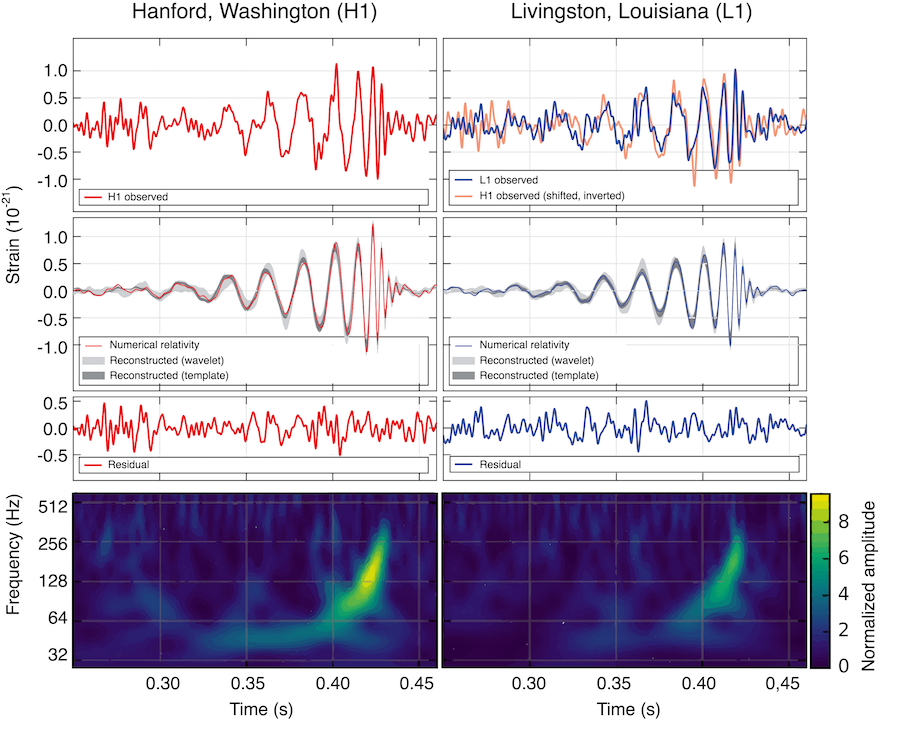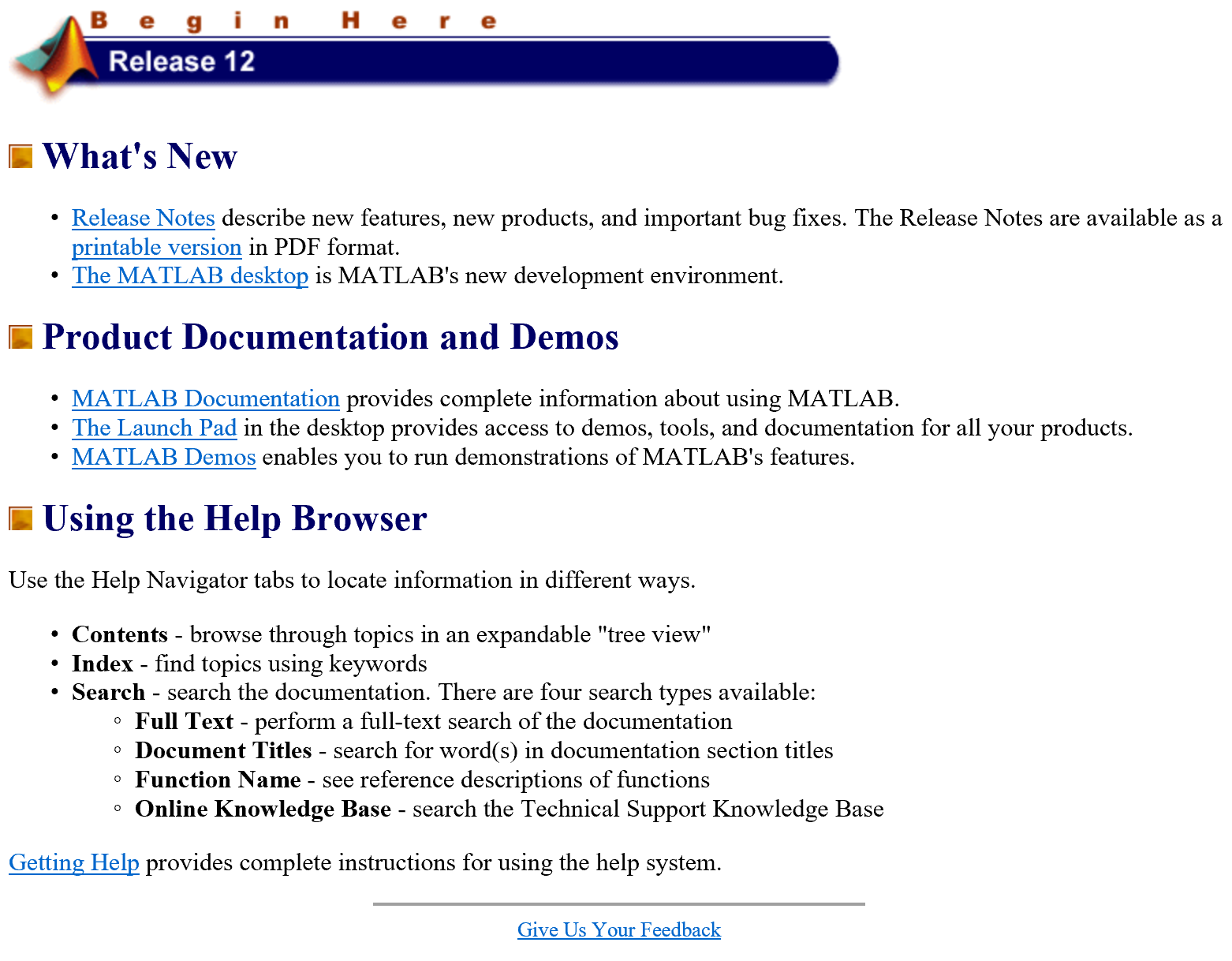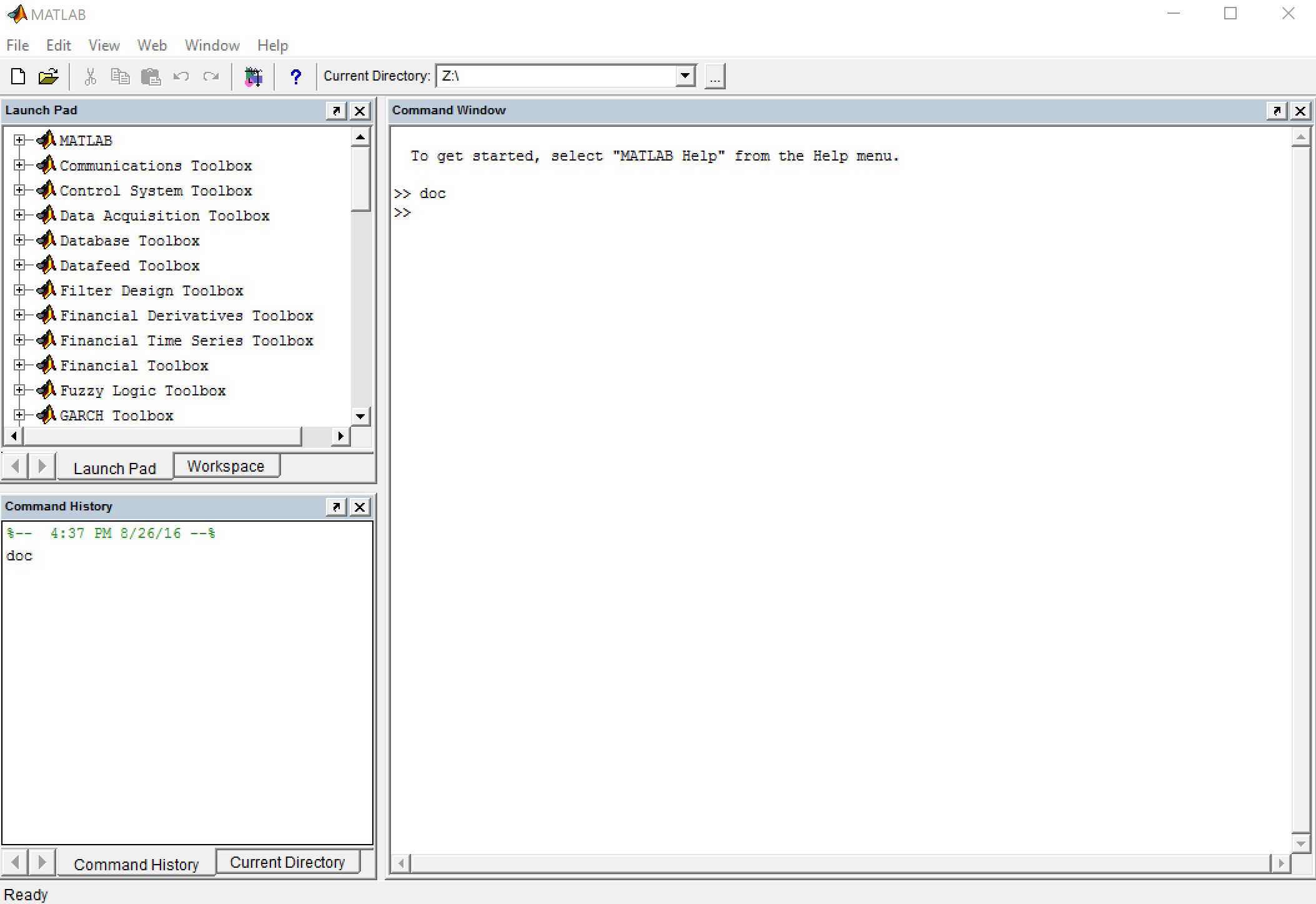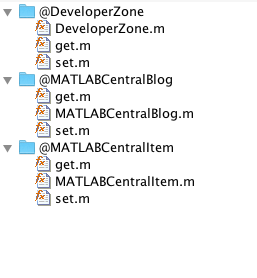# Throwback Thursday: Remembering the olden times

You may have heard some buzz lately about how MATLAB Central is celebrating its quinceañera. Indeed the event is worth a fiery fiesta, and you should definitely check out all of the activities MATLAB Central is hosting to celebrate. There are games, prizes, and all sorts of shenanigans to procrastinate with. You only get one 15 year birthday, and we are trying to make it a good time.

Just thinking about this milestone of the MATLAB web community takes me way back to the wee beginnings of this very blog. Just think of the history! Just two days after this blog started Tom Brady got accused of deflating footballs in the #deflategate debacle. Sheesh, can you believe that? That seems like it only happened last year. In fact, it's amazing I know, but this blog started before any of the following events:

We obtained high resolution photos from a close encounter with Pluto.Image credit: Wikipedia

The Golden State Warriors had their record breaking 73-win season.Image credit: Wikipedia

The internet went cray cray twice over on the same day due to llama drama and a little blue and black dress (yes, I was always on team blue/black!).Image credit: Wikipedia

LIGO detected gravitational waves.Image credit: Wikipedia

SpaceX had an amazing landing of the Falcon 9 rocket.Image credit: Wikipedia

Reality Check

Can you believe it has been that long?

OK, so maybe Developer Zone hasn't really been around that long. In dog years, MATLAB Central is 95 years older than this here humble baby of a blog. Well, if I can't reminisce about the blog, perhaps I can reminisce about MATLAB! I certainly was a MATLAB user 15 years ago. In fact I was a MATLAB user 20 years ago as it was the first programming language I learned as a freshman upstart in mechanical engineering. So hey, 5 years into my illustrious MATLAB career and I was designing controllers and analyzing vibrations in graduate school. That's when MATLAB Central was born.

When MATLAB Central started the version of MATLAB was R12. Take a look at the documentation landing page, we had a brand spankin new feature called "The MATLAB Desktop".Speakin of, let's take a look at the desktop:Wow! Takes me right back to my school days.

Well, given I like to highlight production software design here and there's not too much to reminisce about the history of this still young blog, I thought it would be fun to follow up on the previous post highlighting some of the syntactic sugar that the MATLAB object system provides and take a peak back at the object system of MATLAB past. It's actually a little recognized fact that MATLAB had an object system in those days. Many people only heard of object oriented programming in MATLAB when R2008a was released and the first version of today's loved object system was introduced.

But no! We did, but perhaps with just slightly less syntactic sugar. How much less you ask? Well, let me show you. First, let's see a simple 3 deep hierarchy of classes in today's MATLAB

classdef MATLABCentralItem

properties
HomeURL = 'https://www.mathworks.com/matlabcentral/';
end

end

classdef MATLABCentralBlog < MATLABCentralItem

properties
Topic = 'MATLAB';
Author = 'Cleve';
end

end

classdef DeveloperZone < MATLABCentralBlog

properties
LastPost = 'Encapsulated Sugar';
end

end



OK, nice. Nothing significant. Just a few files containing some classes with just a few properties. How did we implement this 15 years ago? First let me show you the folder structure:Three classes? Three folders. You wanna set and get properties? You gotta implement the set and get methods. How were they implemented? First lets look at the base class:

MATLABCentralItem Constructor

function item = MATLABCentralItem

item.HomeURL = 'https://www.mathworks.com/matlabcentral/';
item = class(item, 'MATLABCentralItem');



MATLABCentralItem Getter

function value = get(item, propName)

switch propName

case 'HomeURL'
value = item.HomeURL;
otherwise
error('Unknown property "%s".', propName);
end



MATLABCentralItem Setter

function item = set(item, varargin)

property_argin = varargin;
while length(property_argin) >= 2
propName = property_argin{1};
value = property_argin{2};
property_argin = property_argin(3:end);
switch propName
case 'HomeURL'
item.HomeURL = value;
otherwise
error('Unknown property "%s".', propName);
end
end



That's looking like a lot of boiler plate. Notice we actually have to implement all of the set/get logic ourselves. How about the first subclass?

MATLABCentralBlog Constructor

function blog = MATLABCentralBlog

superclassObj = MATLABCentralItem;
superclassObj = set(superclassObj,'HomeURL', 'https://blogs.mathworks.com/');

blog.Topic = 'MATLAB';
blog.Author = 'Cleve';
blog = class(blog, 'MATLABCentralBlog', superclassObj);



MATLABCentralBlog Getter

function value = get(blog, propName)

switch propName
case 'Topic'
value = blog.Topic;
case 'Author'
value = blog.Author;
case 'HomeURL'
value = get(blog.MATLABCentralItem, 'HomeURL');
otherwise
error('Unknown property "%s".', propName);
end



MATLABCentralBlog Setter

function blog = set(blog, varargin)

property_argin = varargin;
while length(property_argin) >= 2
propName = property_argin{1};
value = property_argin{2};
property_argin = property_argin(3:end);
switch propName
case 'Topic'
blog.Topic = value;
case 'Author'
blog.Author = value;
case 'HomeURL'
blog.MATLABCentralItem = set(blog.MATLABCentralItem, 'HomeURL', value);
otherwise
error('Unknown property "%s".', propName);
end
end



That's not looking better. We have to reimplement all of the base class property handling too! (Before you chime in, I know there are some ways to design a system around this, but I am having fun here).

DeveloperZone Constructor

function dZone = DeveloperZone

superclassObj = MATLABCentralBlog;
superclassObj = set(superclassObj, 'HomeURL', 'https://blogs.mathworks.com/developer/');
superclassObj = set(superclassObj, 'Topic', 'Production software with MATLAB');
superclassObj = set(superclassObj, 'Author', 'Andy');

dZone.LastPost = 'Encapsulated Sugar';
dZone = class(dZone, 'DeveloperZone', superclassObj);



DeveloperZone Getter

function value = get(dZone, propName)

switch propName
case 'LastPost'
value = dZone.LastPost;
case 'Topic'
value = get(dZone.MATLABCentralBlog, 'Topic');
case 'Author'
value = get(dZone.MATLABCentralBlog, 'Author');
case 'HomeURL'
value = get(dZone.MATLABCentralBlog, 'HomeURL');
otherwise
error('Unknown property "%s".', propName);
end



DeveloperZone Setter

function dZone = set(dZone, varargin)

property_argin = varargin;
while length(property_argin) >= 2
propName = property_argin{1};
value = property_argin{2};
property_argin = property_argin(3:end);
switch propName
case 'LastPost'
dZone.LastPost = value;
case 'Topic'
dZone.MATLABCentralBlog = set(dZone.MATLABCentralBlog, 'Topic', value);
case 'Author'
dZone.MATLABCentralBlog = set(dZone.MATLABCentralBlog, 'Author', value);
case 'HomeURL'
dZone.MATLABCentralBlog = set(dZone.MATLABCentralBlog, 'HomeURL', value);
otherwise
error('Unknown property "%s".', propName);
end
end



Whew! My left hand is tired from all that copy and paste. Interestingly, when I was putting together that example I had a bug in the setters where I used length(property_argin) > 2 instead of length(property_argin) >= 2 and none of the set operations were working as a result. I am so glad all of these mechanics are just handled correctly by today's system.

While it is fun to look back and chuckle at our funny clothes and hairstyles (and syntaxes) from long ago, I am actually quite proud of MATLAB when looking back it this. The fact is even though this is much easier nowadays it was a big deal at that time to be able to create objects in the powerful technical computing environment of yesteryear that was MATLAB R12. That said, boy is it a breath of fresh air to see all of the progress we have made since then.

Does it work?

d = DeveloperZone
author = get(d,'Author')
topic = get(d,'Topic')
url = get(d,'HomeURL')
lastPost = get(d,'LastPost')

d =

DeveloperZone object: 1-by-1

author =

Andy

topic =

Production software with MATLAB

url =

https://blogs.mathworks.com/developer/

lastPost =

Encapsulated Sugar



How about setting properties?

d = set(d,'LastPost','Throwback Thursday')
lastPost = get(d,'LastPost')

d =

DeveloperZone object: 1-by-1

lastPost =

Throwback Thursday



What's my name? Of course it works. Do I recommend that you use it for today's code? No. No, I do not.

Did you ever use the older style of object oriented programming? Any stories to tell? Chime in below.

Also, don't forget to head on over to the celebration, MATLAB Central will be sad if you don't show up to the party.

Published with MATLAB® R2016a

|